Courses

# Electric Field MCQ Level - 1

## 25 Questions MCQ Test | Electric Field MCQ Level - 1

Description
This mock test of Electric Field MCQ Level - 1 for Class 12 helps you for every Class 12 entrance exam. This contains 25 Multiple Choice Questions for Class 12 Electric Field MCQ Level - 1 (mcq) to study with solutions a complete question bank. The solved questions answers in this Electric Field MCQ Level - 1 quiz give you a good mix of easy questions and tough questions. Class 12 students definitely take this Electric Field MCQ Level - 1 exercise for a better result in the exam. You can find other Electric Field MCQ Level - 1 extra questions, long questions & short questions for Class 12 on EduRev as well by searching above.
QUESTION: 1

Solution:
QUESTION: 2

Solution:
QUESTION: 3

### An electric dipole is placed at an angle of 300 to a nonuniform electric field. The dipole will experience

Solution:
QUESTION: 4

An electric dipole of moment p is placed at the origin along the x-axis. The electric field at a point P, whose position vector makes an angle θ with the x-axis, will make an angle.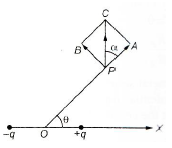Solution:
QUESTION: 5

An electric dipole is situated in an electric field of uniform intensity E whose dipole moment is p and moment of inertia is I. If the dipole is displaced slightly from the equilibrium position, then the angular frequency of its oscillations is

Solution:
QUESTION: 6

Two particles of masses in the ratio 1 : 2 with charges in the ratio 1 : 1, are placed at rest in a uniform electric field. They are relased and allowed to move for the same time. The ratio of their kinetic energies will be finally

Solution:
QUESTION: 7

An α particle is situated in an electric field of strength 15 × 104 NC-1. Force acting on it is

Solution:
QUESTION: 8

A point charge of 100 μC is placed at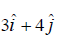m. Find the electric field intensity due to this charge at a point located at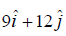m.

Solution:
QUESTION: 9

An oil drop, carrying six electronic charges and having a mass of 1.6 × 10-12 g, falls with some terminal velocity in a medium. What magnitude of vertical electric field is required to make the drop move upwards with the same speed as it was formerly moving downwards with? Ignore buoyancy.

Solution:
QUESTION: 10

Five point charges, +q each, are placed at the five vertices of a regular hexagon. The distance of centre of the hexagon from any of the vertices is a. The electric field at the centre of the hexagon is

Solution:
QUESTION: 11

A ring of charge with radius 0.5 m has 0.002 π m gap. If the ring carries a charge of +1 C, the electric field at the centre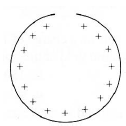Solution:
QUESTION: 12

If an electron has an initial velocity in a direction different from that of a uniform electric field, the path of the electron is

Solution:
QUESTION: 13

A point charge q1 is moved along a circular path of radius r in the electric field of another point charge q2 at the centre of the path. The work done by the electric field on the charge q1 in half revolution is

Solution:
QUESTION: 14

Four identical charges Q are fixed at the four corners of a square of side a. The electric field at a point P located symmetrically at a distance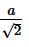from the centre of the square is

Solution:
QUESTION: 15

A thin glass rod is bent into a semicircle of radius r. A charge +Q is uniformly distributed along the upper half and charge -Q is uniformly distributed along the lower half. The electric field E at P, the centre of the semicircle is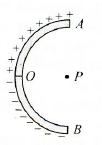Solution:
QUESTION: 16

Find the electric field vector at P (a, a, a) due to three infinitely long lines of charges along the x, y and z axes respectively. The charge density i.e,. charge per unit length of each wire is λ.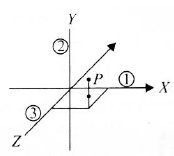Solution:
QUESTION: 17

The unit of electric field is not equivalent to

Solution:
QUESTION: 18

Electric intensity at a point varies as r-1 for

Solution:
QUESTION: 19

The force between two short electric dipole separated by a distance r varies as

Solution:
QUESTION: 20

An electric field can deflect

Solution:
QUESTION: 21

The magnitude of electric field strength E such that an electron placed in it would experience an electrical force equal to its weight is given by

Solution:
QUESTION: 22

A thin conducting ring of radius R is given a charge + Q. The electric field at the centre O of the ring due to charge on the part AKB is E. The electric field at the centre due to the charge on the part ACDB of the ring is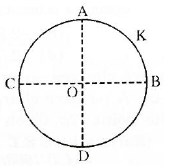Solution:
QUESTION: 23

If electric field is uniform, then the electric lines of forces are

Solution:
QUESTION: 24

Six charges +Q each are placed at the corners of a regular hexagon of side α . The electric field at the centre of hexagon is :

Solution:
QUESTION: 25

The electric dipole is situated in an electric field as shown in fig. The dipole and electric field are both in the plane of the paper. The dipole is rotated about an axis perpendicular to plane of paper passing through A in anticlockwise direction. If the angle of rotation ( θ ) is measured with respect to the direction of electric field, then the torque (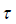) experienced by the dipole for different values of the angle of rotationq will be represented in fig. (b) by curve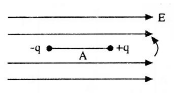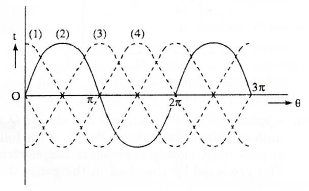Solution: